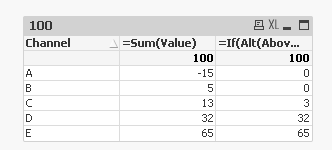# QlikView App Development

Discussion Board for collaboration related to QlikView App Development.

Announcements
Leverage your QlikView investment to modernize BI – see how! Join Group
cancel
Showing results for
Did you mean:
HighlightedPartner

## Calculation based on previous number

Hi,

Kindly find below table and required otuput. This needs to be achieved in frontend. Please assist.

 Channel Value % Calculation ! Required Output A -15 If(Negative then 0) 0 B 5 -15+5 = -10 (Again negative then 0) 0 C 13 -10 + 13 = 3 3 D 32 32 E 65 65 Total 100 100

Regards

Sumeet

1 Solution

Accepted Solutions
HighlightedMVP

## Re: Calculation based on previous number

May be try this

=If(Alt(Above(RangeSum(Above(Sum(Value), 0, RowNo()))), -1) < 0, RangeMax(RangeSum(Above(Sum(Value), 0, RowNo())), 0), Sum(Value))5 Replies
HighlightedCreator II

## Re: Calculation based on previous number

Hi, try this,

tmp:

Channel, Value

A, -15

B, 5

C, 13

D, 32

E, 65

];

tmp1:

Channel,

Value,

if(RowNo() = 1, Value,

Peek(x) + Value)  as x

Resident tmp;

DROP Table tmp;

tmp2:

*,

if(y < 0, 0, y) as z;

*,

if(Peek(x) > 0, Value, x) as y

Resident tmp1;

DROP Table tmp1;

DROP Fields x,y;

Reguards

HighlightedCreator III

## Re: Calculation based on previous number

try this

create one straight table and

expression 1 as sum(value)

expression 2 as =if(RangeSum(Above(Value,0,2))<0,'0',RangeSum(Above(Value,0,2)))

HighlightedMVP

## Re: Calculation based on previous number

May be try this

=If(Alt(Above(RangeSum(Above(Sum(Value), 0, RowNo()))), -1) < 0, RangeMax(RangeSum(Above(Sum(Value), 0, RowNo())), 0), Sum(Value))HighlightedCreator III

## Re: Calculation based on previous number

greatHighlightedPartner

## Re: Calculation based on previous number

Great Sunny..!!! Thanks a ton

Regards

Sumeet